NEET  >  NCERT Solutions: Mechanical Properties of Solids

# Mechanical Properties of Solids NCERT Solutions - Physics Class 11 - NEET

NCERT QUESTION

(Mechanical Properties Of Solids)

Question 9.1: A steel wire of length 4.7 m and cross-sectional area 3.0 x 10–5 m2 stretches by the same amount as a copper wire of length 3.5 m and cross-sectional area of 4.0 x 10–5 m2 under a given load. What is the ratio of the Young’s modulus of steel to that of copper?

ANSWER : - Length of the steel wire, L1 = 4.7 m

Area of cross-section of the steel wire, A1 = 3.0 x 10–5 m2

Length of the copper wire, L2 = 3.5 m

Area of cross-section of the copper wire, A2 = 4.0 x 10–5 m2

Change in length = ΔL1 = ΔL2 = ΔL

Force applied in both the cases = F

Young’s modulus of the steel wire: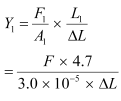.....(i)

Young’s modulus of the copper wire: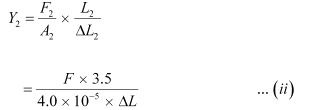Dividing (i) by (ii), we get: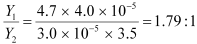The ratio of Young’s modulus of steel to that of copper is 1.79 : 1.

Question 9.2:  Figure 9.11 shows the strain-stress curve for a given material. What are (a) Young’s modulus and (b) approximate yield strength for this material?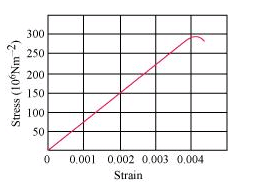ANSWER : - (a) It is clear from the given graph that for stress 150 x 106 N/m2, strain is 0.002.

∴Young’s modulus, Y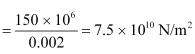Hence, Young’s modulus for the given material is 7.5 x1010 N/m2.

(b) The yield strength of a material is the maximum stress that the material can sustain without crossing the elastic limit.

It is clear from the given graph that the approximate yield strength of this material is    300x106 Nm/2 or 3 x 108 N/m2.

Question 9.3: The stress-strain graphs for materials A and B are shown in Fig. 9.12.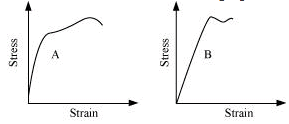The graphs are drawn to the same scale.

(a) Which of the materials has the greater Young’s modulus?

(b) Which of the two is the stronger material?

ANSWER : - (a) A (b) A

(a) For a given strain, the stress for material A is more than it is for material B, as shown in the two graphs.

Young’s modulus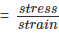For a given strain, if the stress for a material is more, then Young’s modulus is also greater for that material. Therefore, Young’s modulus for material A is greater than it is for material B.

(b) The amount of stress required for fracturing a material, corresponding to its fracture point, gives the strength of that material. Fracture point is the extreme point in a stress-strain curve. It can be observed that material A can withstand more strain than material B. Hence, material A is stronger than material B.

Question 9.4: Read the following two statements below carefully and state, with reasons, if it is true or false.

(a) The Young’s modulus of rubber is greater than that of steel;

(b) The stretching of a coil is determined by its shear modulus.

ANSWER : - (a) False (b) True

(a) For a given stress, the strain in rubber is more than it is in steel.

Young’s modulus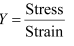For a constant stress: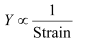Hence, Young’s modulus for rubber is less than it is for steel.

(b) Shear modulus is the ratio of the applied stress to the change in the shape of a body. The stretching of a coil changes its shape. Hence, shear modulus of elasticity is involved in this process.

Question 9.5: Two wires of diameter 0.25 cm, one made of steel and the other made of brass are loaded as shown in Fig. 9.13. The unloaded length of steel wire is 1.5 m and that of brass wire is 1.0 m. Compute the elongations of the steel and the brass wires.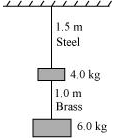ANSWER : - Elongation of the steel wire = 1.49 x 10–4 m

Elongation of the brass wire = 1.3 x 10–4 m

Diameter of the wires, d = 0.25 m

Hence, the radius of the wires,   = 0.125 cm

Length of the steel wire, L1 = 1.5 m

Length of the brass wire, L2 = 1.0 m

Total force exerted on the steel wire:

F1 = (4 6) g = 10 x 9.8 = 98 N

Young’s modulus for steel: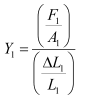Where,

ΔL1 = Change in the length of the steel wire

A1 = Area of cross-section of the steel wire

Young’s modulus of steel, Y1 = 2.0 x 1011 Pa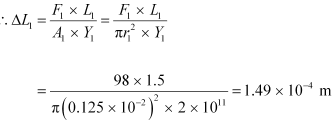Total force on the brass wire:

F2 = 6 x 9.8 = 58.8 N

Young’s modulus for brass: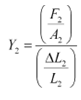Where,
ΔL2 = Change in length
A2 = Area of cross-section of the brass wire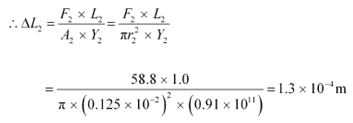Elongation of the steel wire = 1.49 x 10–4 m

Elongation of the brass wire = 1.3 x 10–4 m

Question 9.6: The edge of an aluminium cube is 10 cm long. One face of the cube is firmly fixed to a vertical wall. A mass of 100 kg is then attached to the opposite face of the cube. The shear modulus of aluminium is 25 GPa. What is the vertical deflection of this face?

ANSWER : - Edge of the aluminium cube, L = 10 cm = 0.1 m

The mass attached to the cube, m = 100 kg

Shear modulus (η) of aluminium = 25 GPa = 25 x 109 Pa

Shear modulus, η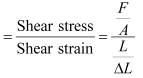Where,

F = Applied force = mg = 100 x 9.8 = 980 N

A = Area of one of the faces of the cube = 0.1 x 0.1 = 0.01 m2

ΔL = Vertical deflection of the cube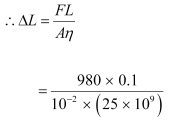= 3.92 x 10–7 m

The vertical deflection of this face of the cube is 3.92 x10–7 m.

Question 9.7: Four identical hollow cylindrical columns of mild steel support a big structure of mass 50,000 kg. The inner and outer radii of each column are 30 cm and 60 cm respectively. Assuming the load distribution to be uniform, calculate the compressional strain of each column.

ANSWER : - Mass of the big structure, M = 50,000 kg

Inner radius of the column, r = 30 cm = 0.3 m

Outer radius of the column, R = 60 cm = 0.6 m

Young’s modulus of steel, Y = 2 x 1011 Pa

Total force exerted, FMg = 50000 x 9.8 N

Stress = Force exerted on a single column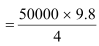= 122500 N

Young’s modulus, Y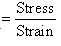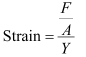Where,

Area, A = π (R2 – r2) = π ((0.6)2 – (0.3)2)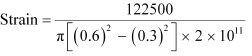= 7.22 x 10–7

Hence, the compressional strain of each column is 7.22 x 10–7.

Question 9.8: A piece of copper having a rectangular cross-section of 15.2 mm x 19.1 mm is pulled in tension with 44,500 N force, producing only elastic deformation. Calculate the resulting strain?

ANSWER : - Length of the piece of copper, l = 19.1 mm = 19.1 x 10–3 m

Breadth of the piece of copper, b = 15.2 mm = 15.2 x 10–3 m

Area of the copper piece:

A = l x b

= 19.1 x 10–3 x 15.2 x 10–3

= 2.9 x 10–4 m2

Tension force applied on the piece of copper, F = 44500 N

Modulus of elasticity of copper, η = 42 x 109 N/m2

Modulus of elasticity, η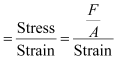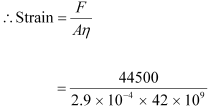= 3.65 x 10–3

Question 9.9: A steel cable with a radius of 1.5 cm supports a chairlift at a ski area. If the maximum stress is not to exceed 108 N m–2, what is the maximum load the cable can support?

ANSWER : - Radius of the steel cable, r = 1.5 cm = 0.015 m

Maximum allowable stress = 108 N m–2

Maximum stress =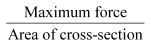∴Maximum force = Maximum stress x Area of cross-section

= 108 x π (0.015)2

= 7.065 x 104 N

Hence, the cable can support the maximum load of 7.065 x 104 N.

Question 9.10: A rigid bar of mass 15 kg is supported symmetrically by three wires each 2.0 m long. Those at each end are of copper and the middle one is of iron. Determine the ratio of their diameters if each is to have the same tension.

ANSWER : - The tension force acting on each wire is the same. Thus, the extension in each case is the same. Since the wires are of the same length, the strain will also be the same.

The relation for Young’s modulus is given as: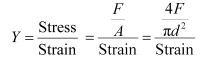Where,

F = Tension force

A = Area of cross-section

d = Diameter of the wire

It can be inferred from equation (i) that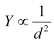Young’s modulus for iron, Y1 = 190 x 109 Pa

Diameter of the iron wire = d1

Young’s modulus for copper, Y2 = 110 x 109 Pa

Diameter of the copper wire = d2

Therefore, the ratio of their diameters is given as: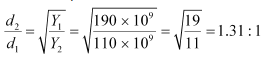Question 9.11: A 14.5 kg mass, fastened to the end of a steel wire of unstretched length 1.0 m, is whirled in a vertical circle with an angular velocity of 2 rev/s at the bottom of the circle. The cross-sectional area of the wire is 0.065 cm2. Calculate the elongation of the wire when the mass is at the lowest point of its path.

ANSWER : - Mass, m = 14.5 kg

Length of the steel wire, l = 1.0 m

Angular velocity, ω = 2 rev/s

Cross-sectional area of the wire, a = 0.065 cm2

Let δl be the elongation of the wire when the mass is at the lowest point of its path.

When the mass is placed at the position of the vertical circle, the total force on the mass is:

Fmg  mlω2

= 14.5 x 9.8 14.5 x 1 x (2)2

= 200.1 N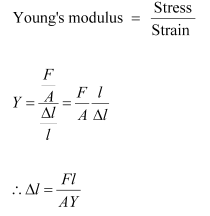Young’s modulus for steel = 2 x 1011 Pa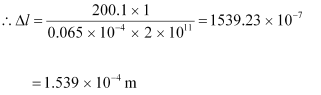Hence, the elongation of the wire is 1.539 x 10–4 m.

Question 9.12: Compute the bulk modulus of water from the following data: Initial volume = 100.0 litre, Pressure increase = 100.0 atm (1 atm = 1.013 x 105 Pa), Final volume = 100.5 litre. Compare the bulk modulus of water with that of air (at constant temperature). Explain in simple terms why the ratio is so large.

ANSWER : - Initial volume, V1 = 100.0l = 100.0 x 10 –3 m3

Final volume, V2 = 100.5 l = 100.5 x10 –3 m3

Increase in volume, ΔVV2 – V1 = 0.5 x 10–3 m3

Increase in pressure, Δp = 100.0 atm = 100 x 1.013 x 105 Pa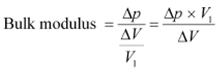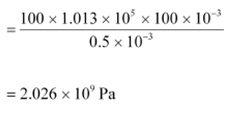Bulk modulus of air = 1.0x 105 Pa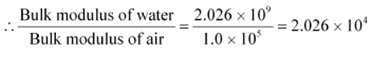This ratio is very high because air is more compressible than water.

Question 9.13: What is the density of water at a depth where pressure is 80.0 atm, given that its density at the surface is 1.03 x 103 kg m–3?

ANSWER : - Let the given depth be h.

Pressure at the given depth, p = 80.0 atm = 80 x 1.01 x 105 Pa

Density of water at the surface, ρ1 = 1.03 x 103 kg m–3

Let ρ2 be the density of water at the depth h.

Let V1 be the volume of water of mass m at the surface.

Let V2 be the volume of water of mass m at the depth h.

Let ΔV be the change in volume.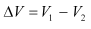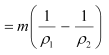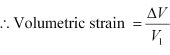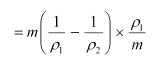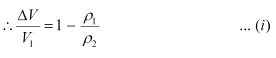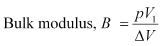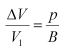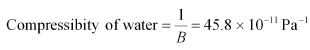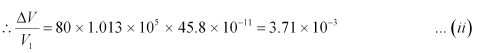For equations (i) and (ii), we get: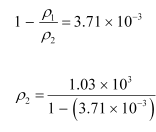Therefore, the density of water at the given depth (h) is 1.034 x 103 kg m–3.

Question 9.14: Compute the fractional change in volume of a glass slab, when subjected to a hydraulic pressure of 10 atm.

ANSWER : - Hydraulic pressure exerted on the glass slab, p = 10 atm = 10 x 1.013 x 105 Pa

Bulk modulus of glass, B = 37 x 109 Nm–2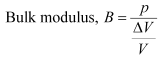where,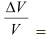Fractional change in volume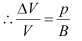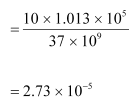Hence, the fractional change in the volume of the glass slab is 2.73 x 10–5.

Question 9.15: Determine the volume contraction of a solid copper cube, 10 cm on an edge, when subjected to a hydraulic pressure of 7.0 x106 Pa.

ANSWER : - Length of an edge of the solid copper cube, l = 10 cm = 0.1 m

Hydraulic pressure, p = 7.0 x106 Pa

Bulk modulus of copper, B = 140 x 109 Pa

Bulk modulus,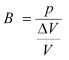Where,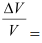Volumetric strain

ΔV- Change in volume
V = Original volume.

Where,

= Volumetric strain

ΔV = Change in volume

V = Original volume.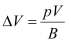Original volume of the cube, V = l3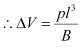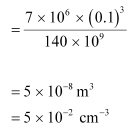Therefore, the volume contraction of the solid copper cube is 5 x 10–2 cm–3.

Question 9.16: How much should the pressure on a litre of water be changed to compress it by 0.10%?

ANSWER : - Volume of water, V = 1 L

It is given that water is to be compressed by 0.10%.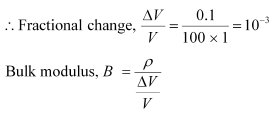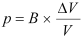Hulk modulus of water.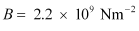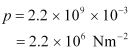Therefore, the pressure on water should be 2.2 x106 Nm–2.

Question 9.17: Anvils made of single crystals of diamond, with the shape as shown in Fig. 9.14, are used to investigate behaviour of materials under very high pressures. Flat faces at the narrow end of the anvil have a diameter of 0.50 mm, and the wide ends are subjected to a compressional force of 50,000 N. What is the pressure at the tip of the anvil?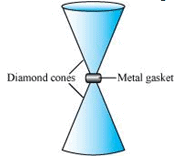ANSWER : - Diameter of the cones at the narrow ends, d = 0.50 mm = 0.5 x 10–3 m

Compressional force, F = 50000 N

Pressure at the tip of the anvil: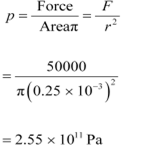Therefore, the pressure at the tip of the anvil is 2.55 x 1011 Pa.

Question 9.18: A rod of length 1.05 m having negligible mass is supported at its ends by two wires of steel (wire A) and aluminium (wire B) of equal lengths as shown in Fig. 9.15. The cross-sectional areas of wires A and B are 1.0 mm2 and 2.0 mm2, respectively. At what point along the rod should a mass m be suspended in order to produce (a) equal stresses and (b) equal strains in both steel and aluminium wires.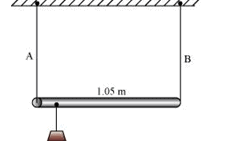ANSWER : - (a) 0.7 m from the steel-wire end

(b) 0.432 m from the steel-wire end

Cross-sectional area of wire Aa1 = 1.0 mm2 = 1.0 x 10–6 m2

Cross-sectional area of wire Ba2 = 2.0 mm2 = 2.0 x 10–6 m2

Young’s modulus for steel, Y1 = 2 x 1011 Nm–2

Young’s modulus for aluminium, Y2 = 7.0 x1010 Nm–2

(a) Let a small mass m be suspended to the rod at a distance y from the end where wire A is attached.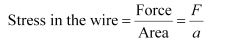If the two wires have equal stresses, then: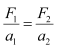Where,

F1 = Force exerted on the steel wire

F2 = Force exerted on the aluminum wire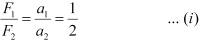The situation is shown in the following figure.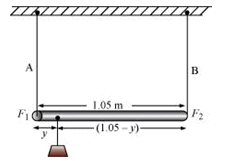Taking torque about the point of suspension, we have: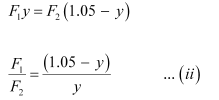Using equations (i) and (ii), we can write: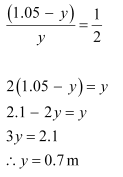In order to produce an equal stress in the two wires, the mass should be suspended at a distance of 0.7 m from the end where wire A is attached.

(b)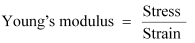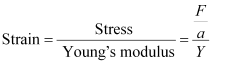If the strain in the two wires is equal, then: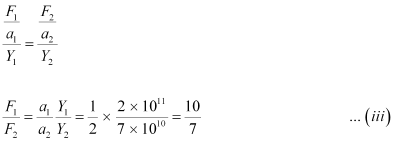Taking torque about the point where mass m, is suspended at a distance y1 from the side where wire A attached, we get:

F1y1F2 (1.05 – y1)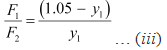Using equations (iii) and (iv), we get: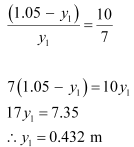In order to produce an equal strain in the two wires, the mass should be suspended at a distance of 0.432 m from the end where wire A is attached.

Question 9.19: A mild steel wire of length 1.0 m and cross-sectional area 0.50 x 10–2 cm2 is stretched, well within its elastic limit, horizontally between two pillars. A mass of 100 g is suspended from the mid-point of the wire. Calculate the depression at the midpoint.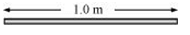Length of the steel wire = 1.0 m

Area of cross-section, A = 0.50 x 10–2 cm2 = 0.50 x 10–6 m2

A mass 100 g is suspended from its midpoint.

m = 100 g = 0.1 kg

Hence, the wire dips, as shown in the given figure.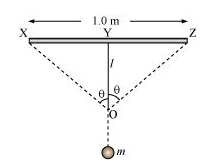Original length = XZ

Depression = l

The length after mass m, is attached to the wire = XO OZ

Increase in the length of the wire:

Δl = (XO OZ) – XZ

Where,

XO = OZ =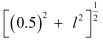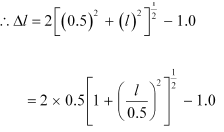Expanding and neglecting higher terms, we get: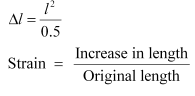Let T be the tension in the wire.

mg = 2T cosθ

Using the figure, it can be written as: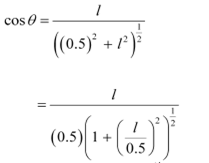Expanding the expression and eliminating the higher terms: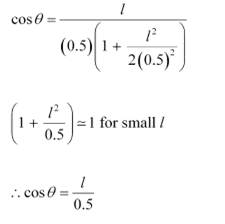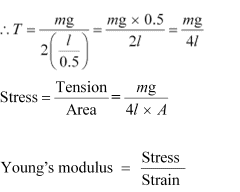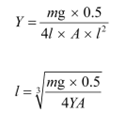Young’s modulus of steel, Y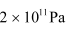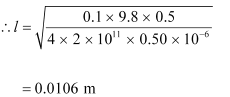Hence, the depression at the midpoint is 0.0106 m.

Question 9.20: Two strips of metal are riveted together at their ends by four rivets, each of diameter 6.0 mm. What is the maximum tension that can be exerted by the riveted strip if the shearing stress on the rivet is not to exceed 6.9 x 107 Pa? Assume that each rivet is to carry one quarter of the load.

ANSWER : - Diameter of the metal strip, d = 6.0 mm = 6.0 x 10–3 m

Maximum shearing stress = 6.9 x 107 Pa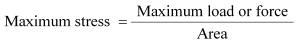Maximum force = Maximum stress x Area

= 6.9 x 107 x π x (r2

= 6.9 x 107 x π x (3 x10–3)2

= 1949.94 N

Each rivet carries one quarter of the load.

∴ Maximum tension on each rivet = 4 x 1949.94 = 7799.76 N

Question 9.21: The Marina trench is located in the Pacific Ocean, and at one place it is nearly eleven km beneath the surface of water. The water pressure at the bottom of the trench is about 1.1 x 108 Pa. A steel ball of initial volume 0.32 m3 is dropped into the ocean and falls to the bottom of the trench. What is the change in the volume of the ball when it reaches to the bottom?

ANSWER : - Water pressure at the bottom, p = 1.1 x 108 Pa

Initial volume of the steel ball, V = 0.32 m3

Bulk modulus of steel, B = 1.6 x 1011 Nm–2

The ball falls at the bottom of the Pacific Ocean, which is 11 km beneath the surface.

Let the change in the volume of the ball on reaching the bottom of the trench be ΔV.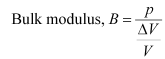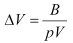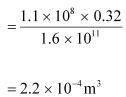Therefore, the change in volume of the ball on reaching the bottom of the trench is 2.2 x 10–4 m3

The document Mechanical Properties of Solids NCERT Solutions | Physics Class 11 - NEET is a part of the NEET Course Physics Class 11.
All you need of NEET at this link: NEET

## FAQs on Mechanical Properties of Solids NCERT Solutions - Physics Class 11 - NEET

 1. What are the mechanical properties of solids?Ans. Mechanical properties of solids refer to the physical characteristics that determine how a solid material responds to external forces or loads. These properties include elasticity, plasticity, stiffness, toughness, strength, and hardness.
 2. What is elasticity in solids?Ans. Elasticity is the ability of a solid material to regain its original shape and size after the applied external force is removed. It is a measure of how much a solid can deform under stress and then return to its original form when the stress is released.
 3. What is plasticity in solids?Ans. Plasticity is the property of a solid material to undergo permanent deformation without breaking or fracturing. In other words, it is the ability of a solid to change its shape and size even after the removal of the applied force.
 4. What is the difference between strength and hardness in solids?Ans. Strength is the ability of a solid material to withstand an applied force without yielding or breaking. It is a measure of the material's resistance to deformation under stress. On the other hand, hardness is the property that determines a material's ability to resist indentation, scratching, or penetration. It is a measure of the material's resistance to localized deformation.
 5. How are the mechanical properties of solids determined?Ans. The mechanical properties of solids are determined through various experimental techniques such as tensile testing, hardness testing, impact testing, and compression testing. These tests help in measuring parameters such as stress, strain, yield strength, ultimate strength, modulus of elasticity, and toughness, which are used to characterize the mechanical behavior of solids.

## Physics Class 11

130 videos|483 docs|210 tests

## Physics Class 11

130 videos|483 docs|210 tests

### How to Prepare for NEET

Read our guide to prepare for NEET which is created by Toppers & the best Teachers
Signup to see your scores go up within 7 days! Learn & Practice with 1000+ FREE Notes, Videos & Tests.
10M+ students study on EduRev
Track your progress, build streaks, highlight & save important lessons and more!(Scan QR code)
Related Searches

,

,

,

,

,

,

,

,

,

,

,

,

,

,

,

,

,

,

,

,

,

;